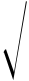# Nanoparticle Carriers Calculator

• Nanoparticle General Parameter Calculator
• Medical Nanoparticle Related Calculator
• Liposome Related Parameter Calculator

Nanoparticle Size Calculator

Small Angle X-ray Scattering (SAXS)

Small angle scattering refers to the coherent scattering phenomenon near the origin (000) node of the reciprocal lattice in diffraction. The scattering angle is on the order of 10-2-10-1 rad. The intensity of the diffracted light is the largest in the direction of the incident light, decreases with the increase of the diffraction angle, and becomes 0 at the angle , and the relationship between and the wavelength λ and the average particle diameter d approximately satisfies the following relationship:

Related formulas

ε0 =

ε0 =

Note: θ is the scattering angle (degrees). Small-angle X-ray scattering (SAXS) technology can study various particles in the range of a few nanometers to hundreds of nanometers. Analysis of small-angle scattering patterns can obtain the long-period structure information of matter, or the shape, scale or mass information of submicron particles (or pores). Small angle scattering is an extremely powerful technique or tool for analyzing the spatial correlation of diffuse objects, such as polymer chains and macromolecules in solution.

View all Biopolymers & Synthetic Polymers Products

X-ray Diffraction

According to X-ray diffraction (XRD) theory, when the grain size is less than 100 nm, the broadening of the diffraction peak becomes significant as the grain size becomes smaller. Considering the absorption effect of the sample and the influence of the structure on the diffraction line shape, the grain size of the sample can be used Debye-Scherrer formula calculation.

Related formulas

d =

Note: d is the grain size, nm; B is the integral width at half maximum, which needs to be converted into radians during the calculation process, rad; θ is the diffraction angle, (°); λ is the X-ray wavelength, nm.

Raman scattering method

Raman scattering (LRS) can measure the average particle size of nanocrystalline grains, and the average particle size is calculated by the following calculator:

 B cm^-1 Δπ ω cm^-1

Related formulas

d = 2πNote: B is a constant (different elements have different values); Δπ is the anisotropy factor; ω is the Raman peak frequency; d is the average particle size path.

Quote Request Form
• Notes: Using the company/organization email address would make it easier to reach you. Thank you.

Services
Fill out the form below